﻿ 一种双优选的半监督回归算法
«上一篇文章快速检索 高级检索

 智能系统学报2019, Vol. 14Issue (4): 689-696  DOI: 10.11992/tis.2018050100

### 引用本文CHENG Kangming, XIONG Weili. A dual-optimal semi-supervised regression algorithm[J]. CAAI Transactions on Intelligent Systems, 2019, 14(4): 689-696. DOI: 10.11992/tis.201805010.### 文章历史

1. 江南大学 物联网工程学院，江苏 无锡 214122;
2. 江南大学 轻工过程先进控制教育部重点实验室，江苏 无锡 214122

A dual-optimal semi-supervised regression algorithm
CHENG Kangming 1, XIONG Weili 1,21. School of Internet of Things Engineering, Jiangnan University, Wuxi 214122, China;
2. Key Laboratory of Advanced Process Control for Light Industry (Ministry of Education), Jiangnan University, Wuxi 214122, China
Abstract: Aiming at the problem that there are few label samples in some industrial processes and that the traditional semi-supervised learning cannot guarantee the accurate prediction of unlabeled samples, a dual-optimal semi-supervised regression algorithm is proposed in this paper. First, in this method, the center of the label-concentrated area is found, and the similarity between unlabeled samples and the center is calculated, and therefore, the unlabeled samples are optimized. At the same time, the labeled samples are selected according to similarity between the unlabeled samples and the center of the dense area. Second, by employing the Gaussian process regression method, an auxiliary learner is established according to the selected labeled sample, and then the labels of the selected unlabeled samples are predicted by the auxiliary learner. Finally, the performance of the main learner is improved with these pseudo-label samples. Through a simulation of the numerical case and the actual debutanizer process, the proposed method is verified to have a good prediction performance when the labeled samples are few.
Key words: unlabeled samples    select    semi-supervised regression    center of sample dense area    similarity    Gaussian process regression    auxiliary learner    main learner    debutanizer process    prediction performance

1 高斯过程回归

GPR是一种基于统计学习理论的非参数概率模型，适合处理高维度、小样本及非线性等数据的建模问题

 $\mu \left( {y^*} \right) = {{{k}}^{\rm{T}}}\left( {{{{x}}^*}} \right){{{K}}^{ - 1}}y$ (1)
 ${\sigma ^2}\left( {{y^*}} \right) = {{C}}\left( {{{{x}}^*},{{{x}}^*}} \right) - {{{k}}^{\rm{T}}}\left( {{{{x}}^*}} \right){{{K}}^{ - 1}}{{k}}\left( {{{{x}}^*}} \right)$ (2)

 ${{k}}\left( {{{{x}}_i},{{{x}}_j}} \right) = {{\upsilon }}\exp \left[ { - \frac{1}{2}{{\sum\limits_{d = 1}^D {{{{\omega }}_d}\left( {{{x}}_i^d - {{x}}_j^d} \right)^2} }}} \right]$ (3)

2 基于双优选的半监督回归策略

2.1 双优选准则

 $d\left( {{{{x}}_i},{{{x}}_j}} \right) = {\rm sqrt}\left[ {{{\left( {{{{x}}_i} - {{{x}}_j}} \right)}^{\rm{T}}}{{{S}}^{ - 1}}\left( {{{{x}}_i} - {{{x}}_j}} \right)} \right]$ (4)
 ${{S}} = \frac{1}{{n - 1}}\sum\limits_{i = 1}^n {\left( {{{{x}}_i} - {\bar{ x}}} \right){{\left( {{{{x}}_i} - {\bar{ x}}} \right)}^{\rm{T}}}}$ (5)
 ${\bar{ x}} = \frac{1}{n}\sum\limits_{i = 1}^n {{{{x}}_i}}$ (6)

2.2 无标签样本筛选

1) $h$ $\leftarrow$ 0

2) for $i$ =1 to 50 do

3) for $j$ = $i$ +1 to 50 do

4) 利用公式(1)~(3)计算两样本间的马氏距离 $d({{{x}}_i},{{{x}}_j})$

5) if ( $d({{{x}}_i},{{{x}}_j})$ < ${\theta _1}$ ) then

6) $h$ $\leftarrow$ $h$ +1

7) End if

8) End for

9) if ( $h$ >1) then

10) ${{A}}$ $\leftarrow$ ${{{x}}_i}$

14) End if

15) $h$ $\leftarrow$ 0

13) End for

14) ${{C}}\left( k \right)$ $\leftarrow$ $\displaystyle\frac{1}{l}\sum\limits_{m = 1}^l {{{A}}\left( {m,k} \right)}$ $l$ ${{A}}$ 中样本数量， $k$ 为样本维度

15) for $i$ =1 to 500 do

16) 利用公式(1)~(3)计算样本与密集区中心 ${{C}}$ 的马氏距离 ${d_i}$

17) if ( ${d_i}$ < ${\theta _3}$ ) then

18) ${{{M}}_1}$ $\leftarrow$ ${{{x}}'_i}$

19) End if

20) End for

2.3 辅学习器建立

1) $h$ $\leftarrow$ 0

2) for $i$ =1 to 50 do

3) for $j$ = $i$ +1 to 50 do

4) 利用公式(1)~(3)计算两样本间的马氏距离 $d({{{x}}_i},{{{x}}_j})$

5) if ( $d({{{x}}_i},{{{x}}_j})$ < ${\theta _2}$ ) then

6) $h$ $\leftarrow$ $h$ +1

7) End if

8) End for

9) if ( $h$ >1) then

10) ${{{A}}_1}$ $\leftarrow$ ${x_i}$

11) ${{{B}}_1}$ $\leftarrow$ ${y_i}$

12) End if

13) $h$ $\leftarrow$ 0

14) End for

15) ${f_1}$ $\leftarrow$ GPR ( ${{{A}}_1}$ , ${{{B}}_1}$ )

2.4 基于双优选的半监督算法流程

1) 根据优选准则1筛选出无标签样本，得到无标签样本集 ${{{M}}_1}$

2) 根据优选准则2选出有标签样本，建立一个更有针对性的辅学习器 ${f_1}$

3) 利用辅学习器 ${f_1}$ 对无标签样本集 ${{{M}}_1}$ 预测其标签，将所得的伪标签样本集 ${{{S}}_1}$ 添加到初始有标签样本集 ${{S}}$ 中，最后再利用GPR方法建立主学习器。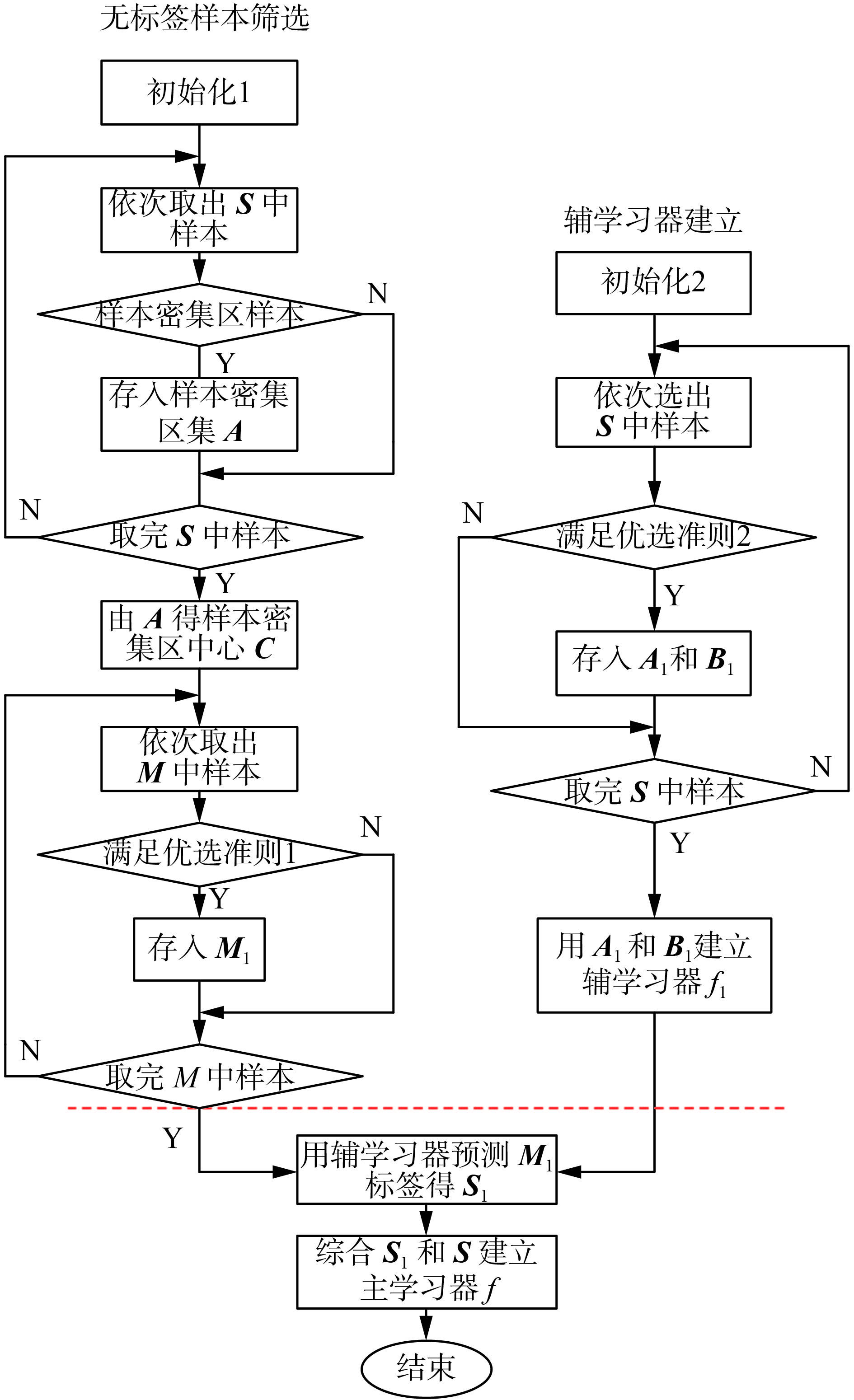Download: 图 1 总体算法步骤 Fig. 1 Overall algorithm steps
3 数值仿真实验

 $\begin{array}{l} \begin{array}{*{20}{c}} {{x_1}\left( {t + 1} \right) = \left( {\displaystyle\frac{{{x_1}\left( t \right)}}{{1 + x_1^2\left( t \right)}} + 1} \right)\sin \left( {{x_2}\left( t \right)} \right)}\\ {{x_2}\left( {t + 1} \right) = {x_2}\left( t \right)\cos \left( {{x_2}\left( t \right)} \right) + {x_1}\left( t \right)\exp \left( { - \displaystyle\frac{{x_1^2\left( t \right) + x_2^2\left( t \right)}}{8}} \right) + }\\ {\displaystyle\frac{{{u^3}\left( t \right)}}{{1 + {u^2}\left( t \right) + 0.5\cos \left( {{x_1}\left( t \right) + {x_2}\left( t \right)} \right)}}}\\ {y\left( t \right) =\displaystyle \frac{{{x_1}\left( t \right)}}{{1 + 0.5\sin \left( {{x_2}\left( t \right)} \right)}} +\displaystyle \frac{{{x_2}\left( t \right)}}{{1 + 0.5\sin \left( {{x_1}\left( t \right)} \right)}} + \varepsilon \left( t \right)} \end{array} \end{array}$

 $y\left( t \right) = f\left( \begin{array}{l} y\left( {t - 1} \right),y\left( {t - 2} \right),y\left( {t - 3} \right) \\ u\left( {t - 1} \right),u\left( {t - 2} \right),u\left( {t - 3} \right) \\ \end{array} \right)$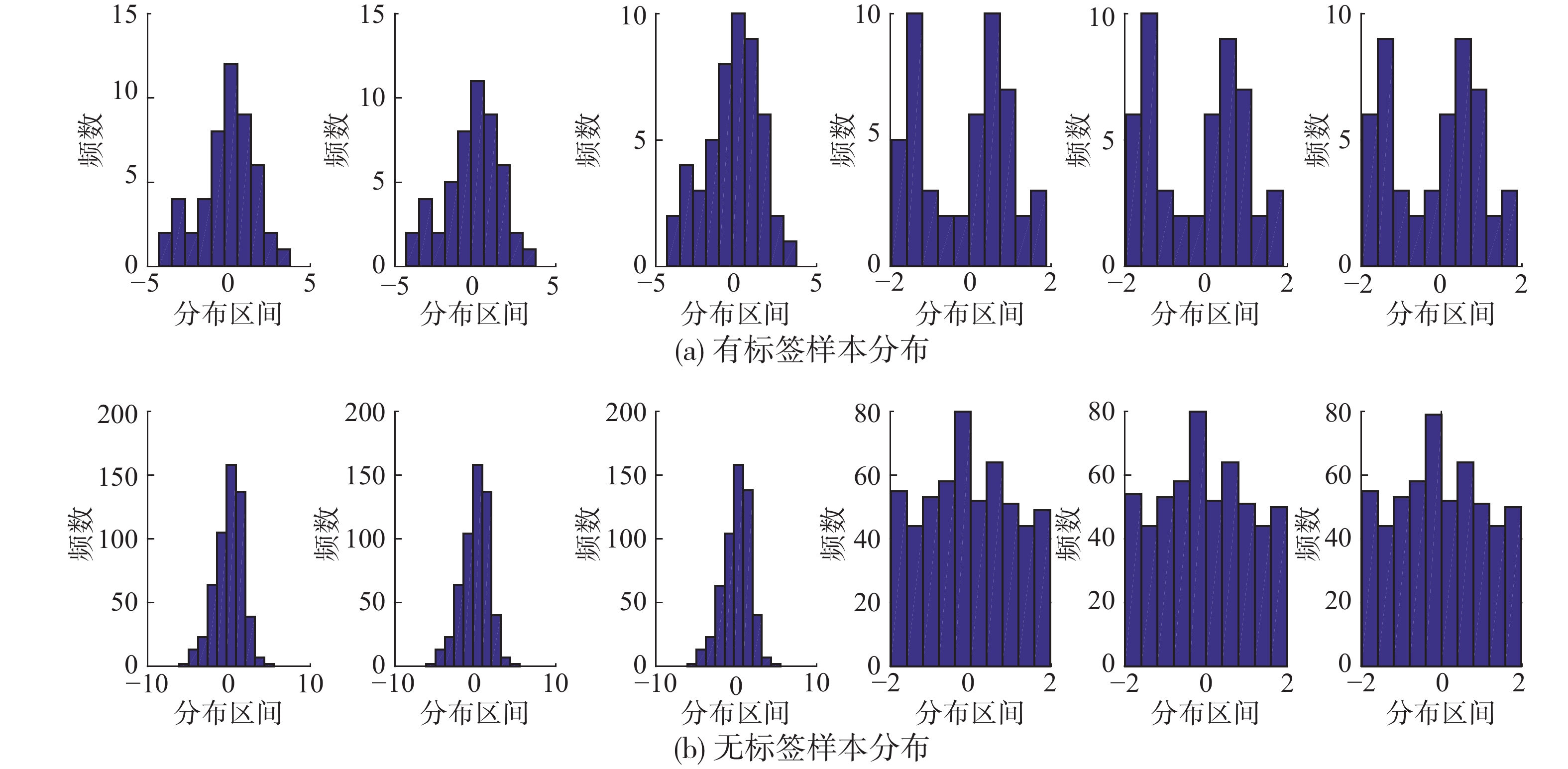Download: 图 2 有标签样本与无标签样本的直方图分布 Fig. 2 Histogram distribution about labeled and unlabeled samples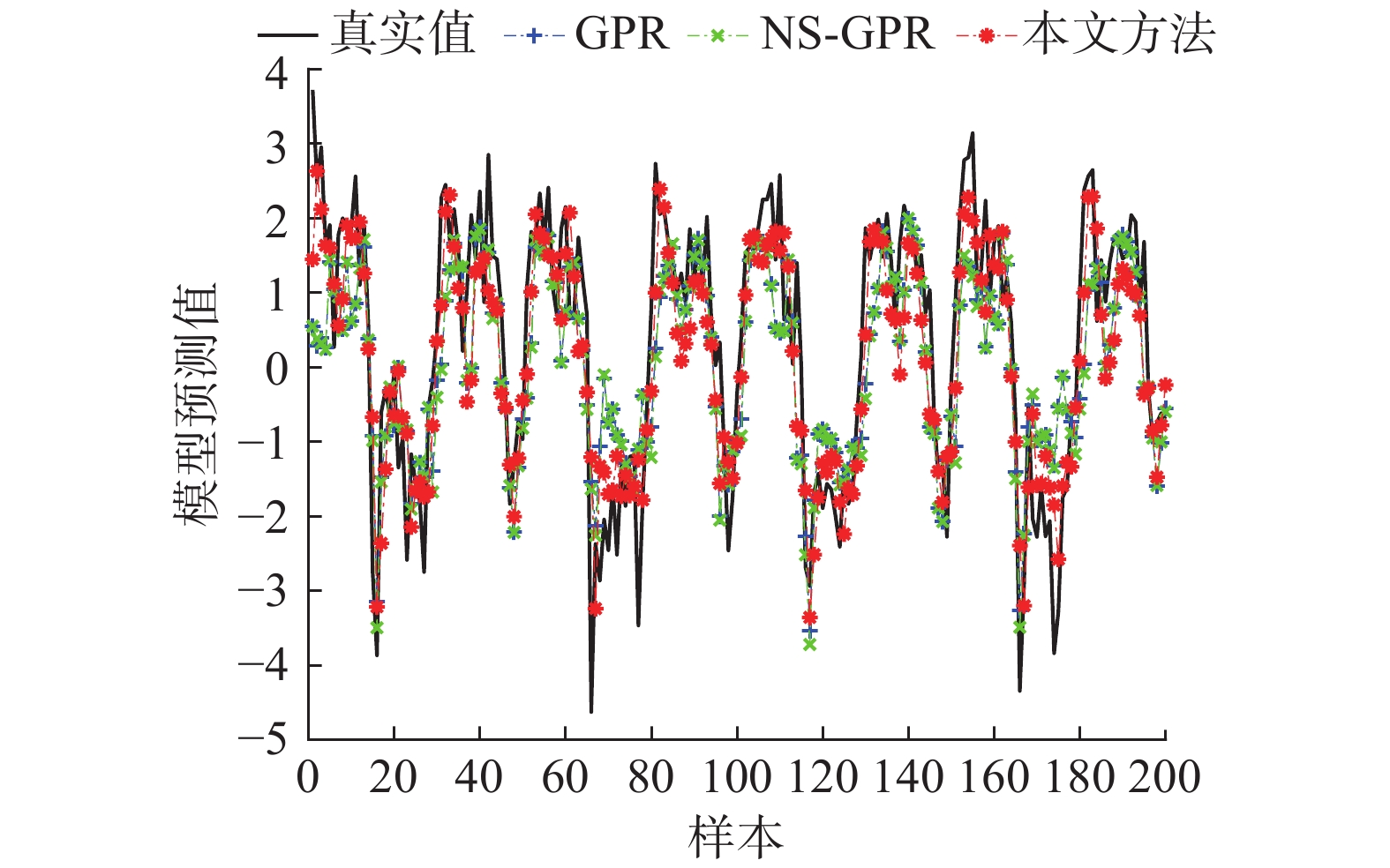Download: 图 3 数值仿真双优选半监督预测效果 Fig. 3 Numerical simulation of double-optimal semi-supervised prediction表 1 数值仿真双优选的拟合性能 Tab.1 The fitting performance of numerical simulation of dual-optimal selection
4 脱丁烷塔仿真实验

1) GPR方法。不利用无标签样本，仅利用已有的有标签样本建立GPR模型，然后测试其对测试样本的跟踪效果。

2) NS-GPR方法。不利用优选准则做筛选，直接对有标签样本建模，获得辅学习器，然后预测无标签样本的标签，进而利用伪标签样本更新主学习器。

3) 第一类单优选半监督GPR(single-optimal semi-supervised GPR，SS-GPRa)。利用优选准则1筛选无标签样本，然后直接对有标签样本建模得到辅学习器，后续过程同方法2)。

4) 第2类单优选半监督GPR(简称SS-GPRb)。首先利用优选准则2筛选有标签样本，然后对其建模得到辅学习器，后续过程同方法2)。

5) 本文方法。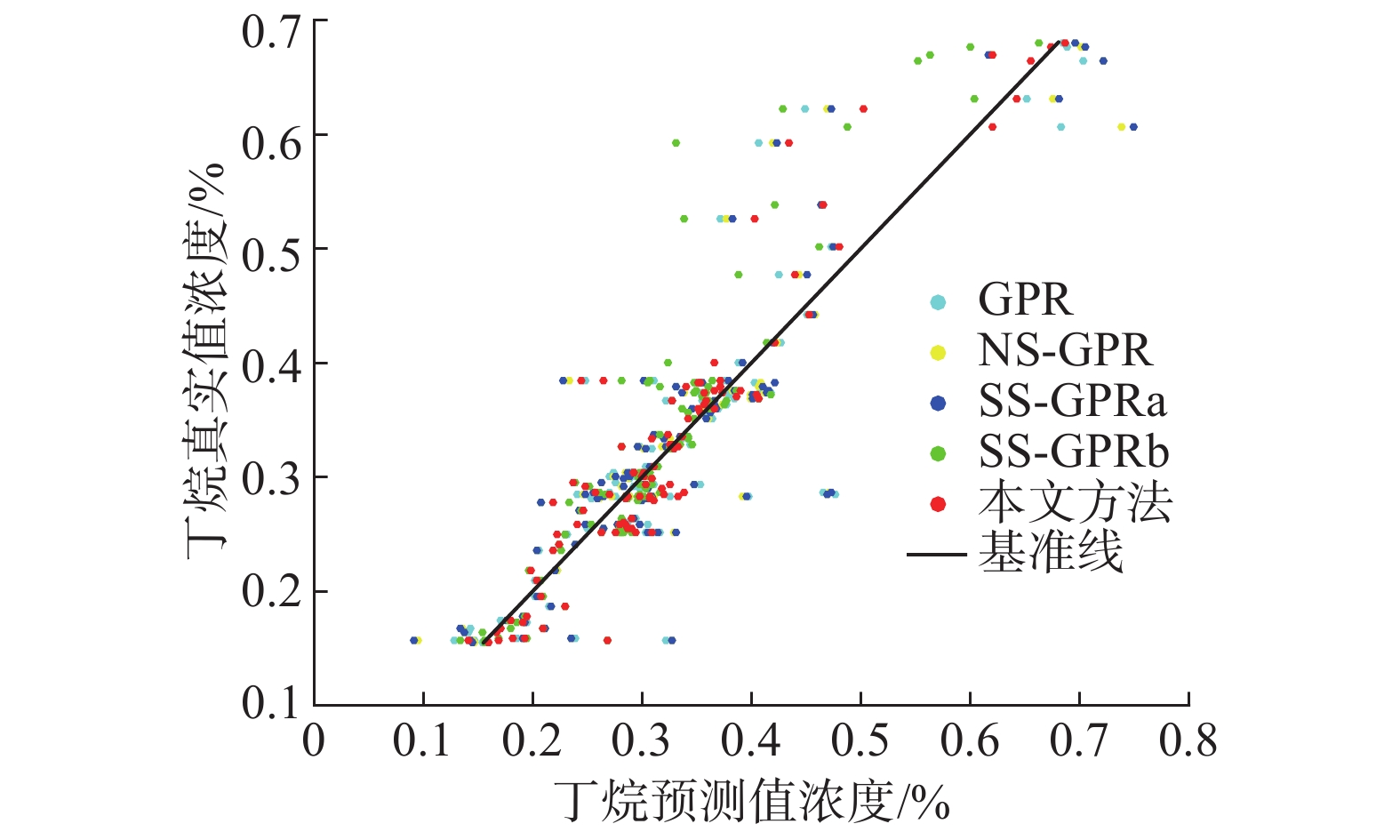Download: 图 4 不同方法的纵向比较 Fig. 4 Longitudinal comparison of different methods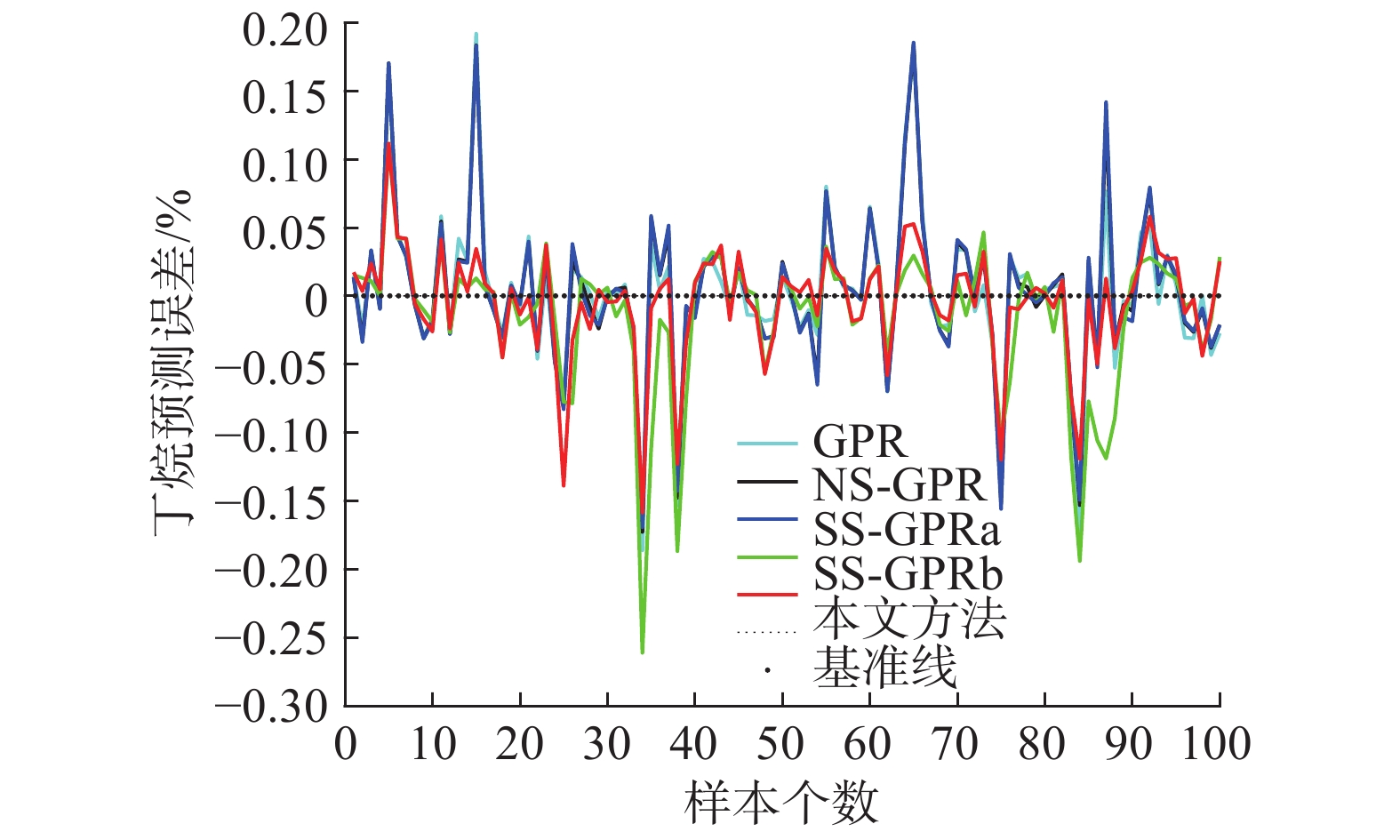Download: 图 5 不同方法的预测误差对比 Fig. 5 Comparison of prediction errors of different methods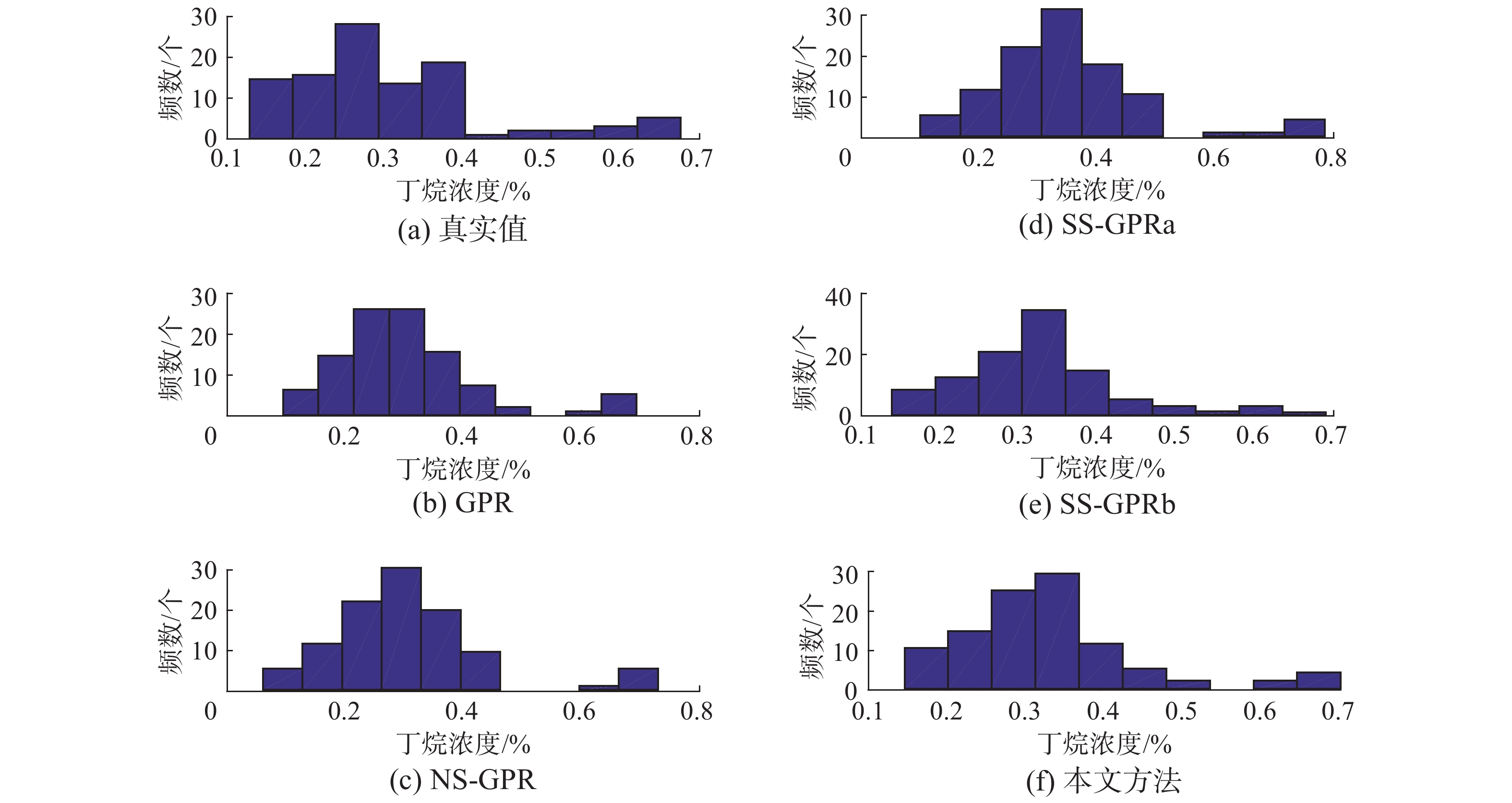Download: 图 6 多种方法预测值与真实值的直方图统计 Fig. 6 Histogram statistics of predicted and real values of various methods表 2 不同模型预测效果对比 Tab.2 Comparison of prediction effects of different models
5 结束语

  周志华. 基于分歧的半监督学习[J]. 自动化学报, 2013, 39(11): 1871-1878. ZHOU Zhihua. Disagreement-based semi-supervised learning[J]. Acta Automatica Sinica, 2013, 39(11): 1871-1878. (0)  ZHOU Zhihua, LI Ming. Semisupervised regression with cotraining-style algorithms[J]. IEEE Transactions on knowledge and data engineering, 2007, 19(11): 1479-1493. DOI:10.1109/TKDE.2007.190644 (0)  姜婷, 袭肖明, 岳厚光. 基于分布先验的半监督FCM的肺结节分类[J]. 智能系统学报, 2017, 12(5): 729-734. JIANG Ting, XI Xiaoming, YUE Houguang. Classification of pulmonary nodules by semi-supervised FCM based on prior distribution[J]. CAAI transactions on intelligent systems, 2017, 12(5): 729-734. (0)  刘建伟, 刘媛, 罗雄麟. 半监督学习方法[J]. 计算机学报, 2015, 38(8): 1592-1617. LIU Jianwei, LIU Yuan, LUO Xionglin. Semi-supervised learning methods[J]. Chinese journal of computers, 2015, 38(8): 1592-1617. (0)  刘杨磊, 梁吉业, 高嘉伟, 等. 基于Tri-training的半监督多标记学习算法[J]. 智能系统学报, 2013, 8(5): 439-445. LIU Yanglei, LIANG Jiye, GAO Jiawei, et al. Semi-supervised multi-label learning algorithm based on Tri-training[J]. CAAI Transactions on intelligent systems, 2013, 8(5): 439-445. (0)  徐蓉, 姜峰, 姚鸿勋. 流形学习概述[J]. 智能系统学报, 2006, 1(1): 44-51. XU Rong, JIANG Feng, YAO Hongxun. Overview of manifold learning[J]. CAAI transactions on intelligent systems, 2006, 1(1): 44-51. (0)  杨剑, 王珏, 钟宁. 流形上的Laplacian半监督回归[J]. 计算机研究与发展, 2007, 44(7): 1121-1127. YANG Jian, WANG Jue, ZHONG Ning. Laplacian semi-supervised regression on a manifold[J]. Journal of computer research and development, 2007, 44(7): 1121-1127. (0)  ZHOU Zhihua, LI Ming. Semi-supervised regression with co-training[C]//Proceedings of the 19th International Joint Conference on Artificial Intelligence. Edinburgh, Scotland, UK, 2005: 908–913. (0)  程玉虎, 冀杰, 王雪松. 基于Help-Training的半监督支持向量回归[J]. 控制与决策, 2012, 27(2): 205-210, 226. CHENG Yuhu, JI Jie, WANG Xuesong. Semi-supervised support vector regression based on Help-Training[J]. Control and decision, 2012, 27(2): 205-210, 226. (0)  盛高斌, 姚明海. 基于半监督回归的选择性集成算法[J]. 计算机仿真, 2009, 26(10): 198-201, 318. SHENG Gaobin, YAO Minghai. An ensemble selection algorithm based on semi - supervised regression[J]. Computer simulation, 2009, 26(10): 198-201, 318. (0)  何志昆, 刘光斌, 赵曦晶, 等. 高斯过程回归方法综述[J]. 控制与决策, 2013, 28(8): 1121-1129, 1137. HE Zhikun, LIU Guangbin, ZHAO Xijing, et al. Overview of Gaussian process regression[J]. Control and decision, 2013, 28(8): 1121-1129, 1137. (0)  熊伟丽, 李妍君, 姚乐, 等. 一种动态校正的AGMM-GPR多模型软测量建模方法[J]. 大连理工大学学报, 2016, 56(1): 77-85. XIONG Weili, LI Yanjun, YAO Le, et al. A dynamically corrected AGMM-GPR multi-model soft sensor modeling method[J]. Journal of Dalian University of Technology, 2016, 56(1): 77-85. (0)  郭帅, 马书根, 李斌, 等. VorSLAM算法中基于多规则的数据关联方法[J]. 自动化学报, 2013, 39(6): 883-894. GUO Shuai, MA Shugen, LI Bin, et al. A data association approach based on multi-rules in VorSLAM[J]. Acta automatica sinica, 2013, 39(6): 883-894. (0)  KNORR E M, NG R T. A unified notion of outliers: properties and computation[C]//Proceedings of the 3rd International Conference on Knowledge Discovery and Data Mining. Newport Beach, CA, USA, 1997: 219–222. (0)  曾静, 王军, 郭金玉. 基于向量相似度的多模型局部建模方法研究[J]. 计算机应用研究, 2012, 29(5): 1631-1633, 1640. ZENG Jing, WANG Jun, GUO Jinyu. Local multi-model method based on similarity of vector[J]. Application research of computers, 2012, 29(5): 1631-1633, 1640. DOI:10.3969/j.issn.1001-3695.2012.05.007 (0)  阮宏镁, 田学民, 王平. 基于联合互信息的动态软测量方法[J]. 化工学报, 2014, 65(11): 4497-4502. RUAN Hongmei, TIAN Xuemin, WANG Ping. Dynamic soft sensor method based on joint mutual information[J]. CIESC journal, 2014, 65(11): 4497-4502. DOI:10.3969/j.issn.0438-1157.2014.11.040 (0)  FORTUNA L, GRAZIANI S, RIZZO A, et al. Soft sensors for monitoring and control of industrial processes[M]. London: Springer, 2007: 229–231. (0)